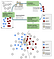# Active learning — Uncertainty Sampling (P3)

The most common strategy that people use to make AI smarter is for the Machine Learning models to tell humans when they are uncertain about a task, and then ask the humans for the correct feedback. In general, unlabeled data that confuses a Machine Learning algorithm will be the most valuable when it is labeled and added to the training data. If the Machine Learning algorithm can already label an item with high confidence it is probably correct already.

## Interpreting Uncertainty in a Machine Learning Model

Uncertainty Sampling is a strategy for identifying unlabeled items that are near a decision boundary in your current Machine Learning model. While it is easy to identify when a model is confident — there is one result with very high confidence — there are many different ways to calculate uncertainty and your choice will depend on your use case and what is the most effective for your particular data.

## Interpreting the scores from your model

almost all Machine Learning models will give you two things:
> A predicted label (or set of predictions)
> A number (or set of numbers) associated with each predicted label.
The numbers are generally interpreted as confidences in the prediction, although this can be more or less true depending on how the numbers are generated. The general principle of Uncertainty Sampling is that if there are mutually exclusive categories with similar confidence, then this is good evidence that the model is confused in the prediction and that a human judgment would be valuable. Therefore, the model will benefit most when it learns to correctly predict the label of an item with an uncertain prediction. For example:

## “Score”, “Confidence”, and “Probability”: Do not trust the name!

Machine Learning libraries will often use the terms “Score”, “Confidence” and “Probability” interchangeably. This is true of open libraries and commercial ones. You might not even find consistency within the same library. Even when the term “probability distribution” is used, it can mean only that the numbers across the predicted labels add up to 100%. It does not necessarily mean that each number reflects the actual model confidence that the prediction is correct. For neural networks, logistic regression, and other types of related Discriminative Supervised Learning Algorithms, it is not the job of the algorithm to know how confident its predictions are: the job of the algorithm is trying “discriminate” between the labels based on the features, hence the name “Discriminative Supervised Learning”. The raw scores from the last layer of a neural network are the network trying to discriminate between the predictions it is making. Depending on the parameters of the model, those raw scores in the final layer can be any real number. So, the scores that come out of these algorithms often need to be converted into something more closely approximating a confidence.

## SoftMax: converting the model output into confidences

The most common models are Neural Networks and Neural Network predictions are almost always converted to a 0–1 range of scores using softmax.

## Algorithms for Uncertainty Sampling

Now that you understand where the confidences in the model predictions come from, you can think about how to interpret the probability distributions in order to find out where your Machine Learning models are most “uncertain”. Uncertainty Sampling is a strategy for identifying unlabeled items that are near a decision boundary in your current Machine Learning model.showing how Uncertainty Sampling as an Active Learning strategy, which over-samples unlabeled items that are closer to the decision boundary

## Least Confidence sampling

The simplest and most common method for uncertainty sampling is to take the difference between 100% confidence and the most confidently predicted label for each item. Let’s refer to the softmax result σ(z) as the probability of the label given the prediction Pθ (y | x). We know that softmax isn’t strictly giving us probabilities, but these are general equations that apply to probability distributions of any sources, not just from softmax

## Margin of Confidence sampling

The most intuitive form of uncertainty sampling is the difference between the two most confident predictions. That is, for the label that the model predicted, how much more confident was it than for the next most confident label? This is defined as:

## Ratio of Confidence sampling

Ratio of confidence is a slight variation on Margin of Confidence, looking at the ratio between the top two scores instead of the difference. It is the best Uncertainty Sampling method to improve your understanding of the relationship between confidence and softmax. To make it a little more intuitive, you can think of the ratio as capturing how many times more likely the first label was than the second most confident:

## Entropy (classification entropy)

One way to look at uncertainty in a set of predictions is by whether you expect to be surprised by the outcome. This is the concept behind entropy: how surprised would you be each of the possible outcomes, relative to their probability? Entropy applied to a probability distribution is multiplying each probability by its own log, and taking the negative sum of those: